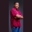Related Tags

swift
or
bitwise
communitycreator

# How to perform bitwise OR operation in SwiftTheodore Kelechukwu Onyejiaku

### Overview

In Swift, performing a bitwise OR operation means adding the binary representation of two decimal values and returning the result in decimal form. We'll need the OR operator |, which takes two operands. The operands here are the numbers we want to get the sum of their bits.

### Syntax

number1 | number2
Syntax for bitwise OR operation in Swift

### Parameters

number1 and number2: These are the number values we want to perform the bitwise OR operation on.

### Return value

The value returned is a decimal representation of the result of adding the bits of number1 and number2.

### Example

// create some values
var num1 = 3
var num2 = 2
var num3 = 100
var num4 = 20
var num5 = 1
var num6 = 0

// perform OR operation
print(num1 | num2) // 3
print(num3 | num4) // 11
print(num5 | num6) // 1
Perform bitwise OR operation in Swift

### Explanation

• Lines 2–7: We create some variables.
• Lines 10–12: Using the OR operator (|), we perform a bitwise OR operation on the values we created, then we print the results to the console.

RELATED TAGS

swift
or
bitwise
communitycreator

CONTRIBUTORTheodore Kelechukwu Onyejiaku
RELATED COURSES

View all Courses

Keep Exploring

Learn in-demand tech skills in half the time